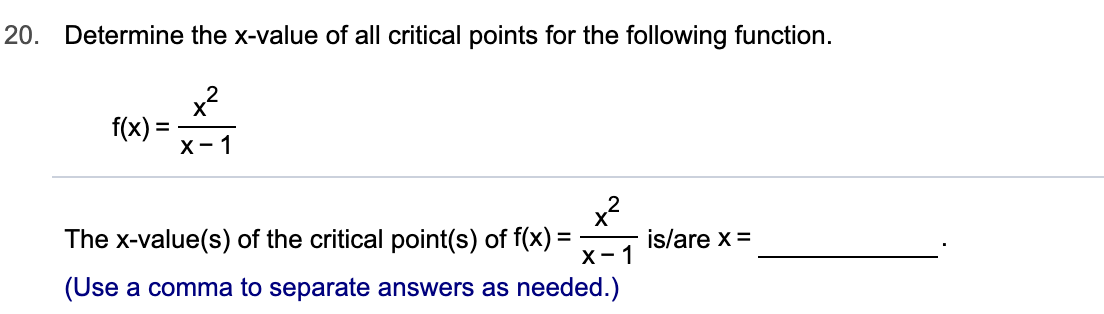# 20. Determine the x-value of all critical points for the following function.f(x)=х- 1xThe x-value(s) of the critical point(s) of f(x)х- 1is/are x ==_(Use a comma to separate answers as needed.)

Question
6 views

Can you help me step by step?help_outlineImage Transcriptionclose20. Determine the x-value of all critical points for the following function. f(x)= х- 1 x The x-value(s) of the critical point(s) of f(x) х- 1 is/are x = =_ (Use a comma to separate answers as needed.) fullscreen
check_circle

Step 1

Given function is

Step 2

To determine x- values of all critical points of the given function

Step 3

Critical points of any function can be determined by e...

### Want to see the full answer?

See Solution

#### Want to see this answer and more?

Solutions are written by subject experts who are available 24/7. Questions are typically answered within 1 hour.*

See Solution
*Response times may vary by subject and question.
Tagged in

### Other